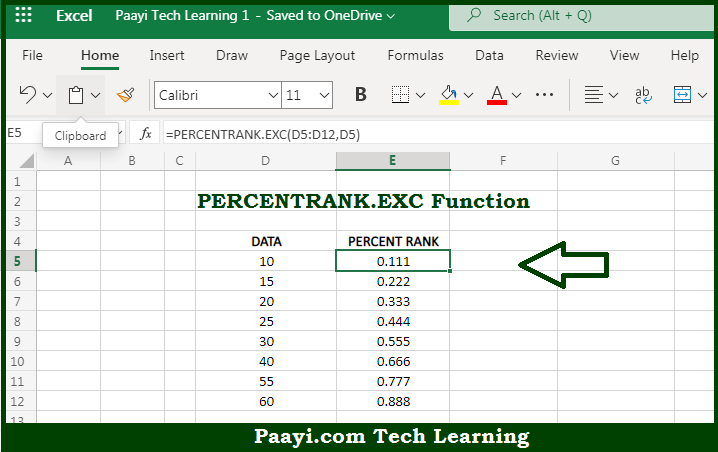# Learn How to Use Microsoft Excel PERCENTRANK.EXC Function

Written by | 0 Comments | 718 Views

In this article, you will learn how to use the Microsoft Excel PERCENTRANK.EXC function and its prime function in Microsoft Excel. You will also get to know the Microsoft Excel PERCENTRANK.EXC function return value and syntax with the help of some example

Microsoft Excel PERCENTRANK.EXC Function

The main purpose of the Microsoft Excel PERCENTRANK.EXC function is to get the percentile rank, exclusive. That implies, with the help of PERCENTRANK.EXC function you can able to return the relative rank of a value in a given data set as a percentage representing how many values are less than or equal to the value. The percentile rank is commonly used as a way to interpret standing in standardized tests. So, with the help of PERCENTRANK.EXC function, you can able to get the percentile rank, exclusive.

Return Value of PERCENTRANK.EXC Function

The return value will be the calculated rank in the decimal value.

Syntax of PERCENTRANK.EXC Function

=PERCENTRANK.EXC(array, x, [significance])

Where the arguments:

• array: This is the data value array.
• x: This is the value to rank.
• significance: This is the number of significant digits in the result. Defaults to 3 (optional).

## How to Use Microsoft Excel PERCENTRANK.EXC Function?So we know that, Microsoft Excel PERCENTRANK.EXC function you can able to get the percentile rank, exclusive. That implies, with the help of PERCENTRANK.EXC function you can able to return the relative rank of a value in a given data set as a percentage representing how many values are less than or equal to the value. The percentile rank is commonly used as a way to interpret standing in standardized tests. So, with the help of PERCENTRANK.EXC function, you can able to get the percentile rank, exclusive.